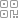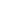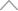6 2 9 1 4 5 3• GPU 渲染的是一个一个的三角形。

• 一个面只要顶点够多，就能生成一个平滑的曲面 ——因此，在低端机可以让三角形【顶点】少一些。

• 动态生成一个 Mesh，是一个平面，并且三角形足够的多。

• 通过一张外部图片【噪声】的信息，来存储云的凹凸信息 ——可以想到，一张图只有黑白灰，越白的地方，让三角形的高度越高，反之亦然。

• 让这张贴图运动【滚动】起来，随着时间的变化，修改获取 UV 的位置信息 ——这样三角形就可以变化了。

• 通过读取多张噪声，或者读取同一张噪声不同位置的地方，叠加起来，就可以获得翻涌的感觉。

https://forum.cocos.org/t/topic/128595

1、准备2、编辑 effect 文件

- vert: general-vs:vert # builtin header

Effect Syntax · Cocos Creator

https://docs.cocos.com/creator/3.3/manual/zh/material-system/effect-syntax.htmlCCProgram my-vs %{

precision highp float;

#include <input-standard>

#include <cc-global>

#include <cc-local-batch>

#include <input-standard>

#include <cc-fog-vs>

in vec4 a_color;

#if HAS_SECOND_UV

in vec2 a_texCoord1;

#endif

out vec3 v_position;

out vec3 v_normal;

out vec3 v_tangent;

out vec3 v_bitangent;

out vec2 v_uv;

out vec2 v_uv1;

out vec4 v_color;

vec4 vert () {

StandardVertInput In;

CCVertInput(In);

mat4 matWorld, matWorldIT;

CCGetWorldMatrixFull(matWorld, matWorldIT);

vec4 pos = matWorld * In.position;

v_position = pos.xyz;

v_normal = normalize((matWorldIT * vec4(In.normal, 0.0)).xyz);

v_tangent = normalize((matWorld * vec4(In.tangent.xyz, 0.0)).xyz);

v_bitangent = cross(v_normal, v_tangent) * In.tangent.w; // note the cross order

v_uv = a_texCoord;

#if HAS_SECOND_UV

v_uv1 = a_texCoord1;

#endif

v_color = a_color;

CC_TRANSFER_FOG(pos);

return cc_matProj * (cc_matView * matWorld) * In.position;

}

}%

CCEffect %{

techniques:

- name: opaque

passes:

- vert: my-vs:vert # builtin header

frag: unlit-fs:frag

properties: &props

mainTexture:    { value: white }

mainColor:      { value: , editor: { type: color } }

- name: transparent

passes:

- vert: general-vs:vert # builtin header

frag: unlit-fs:frag

blendState:

targets:

- blend: true

blendSrc: src_alpha

blendDst: one_minus_src_alpha

blendSrcAlpha: src_alpha

blendDstAlpha: one_minus_src_alpha

properties: *props

}%

3、绑定 effect 到材质上4、创建 Plane 并应用材质5、准备噪声贴图CCProgram unlit-fs %{

precision highp float;

#include <output>

#include <cc-fog-fs>

in vec2 v_uv;

uniform sampler2D mainTexture;

uniform Constant {

vec4 mainColor;

};

vec4 frag () {

vec4 col = mainColor * texture(mainTexture, v_uv + 0.5);

CC_APPLY_FOG(col);

return CCFragOutput(col);

}

}%6、修改顶点着色器uniform sampler2D mainTexture;

vec4 vert () {

StandardVertInput In;

CCVertInput(In);

mat4 matWorld, matWorldIT;

CCGetWorldMatrixFull(matWorld, matWorldIT);

vec4 p = In.position;

float y = texture(mainTexture, a_texCoord).x;

p.y = y;

vec4 pos = matWorld * p;

v_position = pos.xyz;

v_normal = normalize((matWorldIT * vec4(In.normal, 0.0)).xyz);

v_tangent = normalize((matWorld * vec4(In.tangent.xyz, 0.0)).xyz);

v_bitangent = cross(v_normal, v_tangent) * In.tangent.w; // note the cross order

v_uv = a_texCoord;

#if HAS_SECOND_UV

v_uv1 = a_texCoord1;

#endif

v_color = a_color;

CC_TRANSFER_FOG(pos);

return cc_matProj * (cc_matView * matWorld) * p;

}7、平滑import { _decorator, Component, utils, primitives, MeshRenderer } from 'cc';

const { ccclass, property } = _decorator;

@ccclass('MyMesh')

export class MyMesh extends Component {

start () {

const renderer = this.node.getComponent(MeshRenderer);

if(!renderer){

return;

}

const plane: primitives.IGeometry = primitives.plane({

width: 10,

length: 10

widthSegments: 100,

lengthSegments: 100,

});

renderer.mesh = utils.createMesh(plane);

}

}8、运动

uniform sampler2D mainTexture;

#include <cc-global>

vec4 vert () {

StandardVertInput In;

CCVertInput(In);

mat4 matWorld, matWorldIT;

CCGetWorldMatrixFull(matWorld, matWorldIT);

vec4 p = In.position;

float y = texture(mainTexture, a_texCoord + cc_time.x * 0.1).x;

p.y = y;

vec4 pos = matWorld * p;

v_position = pos.xyz;

v_normal = normalize((matWorldIT * vec4(In.normal, 0.0)).xyz);

v_tangent = normalize((matWorld * vec4(In.tangent.xyz, 0.0)).xyz);

v_bitangent = cross(v_normal, v_tangent) * In.tangent.w; // note the cross order

v_uv = a_texCoord;

#if HAS_SECOND_UV

v_uv1 = a_texCoord1;

#endif

v_color = a_color;

CC_TRANSFER_FOG(pos);

return cc_matProj * (cc_matView * matWorld) * p;

}

}%9、颜色

CCProgram my-vs %{

precision highp float;

#include <input-standard>

#include <cc-global>

#include <cc-local-batch>

#include <input-standard>

#include <cc-fog-vs>

in vec4 a_color;

#if HAS_SECOND_UV

in vec2 a_texCoord1;

#endif

out vec3 v_position;

out vec3 v_normal;

out vec3 v_tangent;

out vec3 v_bitangent;

out vec2 v_uv;

out vec2 v_uv1;

out vec4 v_color;

uniform sampler2D mainTexture;

#include <cc-global>

vec4 vert () {

StandardVertInput In;

CCVertInput(In);

mat4 matWorld, matWorldIT;

CCGetWorldMatrixFull(matWorld, matWorldIT);

vec4 p = In.position;

vec4 baseColor0 = texture(mainTexture, a_texCoord + cc_time.x * 0.1);

p.y = baseColor0.x;

vec4 pos = matWorld * p;

v_position = pos.xyz;

v_normal = normalize((matWorldIT * vec4(In.normal, 0.0)).xyz);

v_tangent = normalize((matWorld * vec4(In.tangent.xyz, 0.0)).xyz);

v_bitangent = cross(v_normal, v_tangent) * In.tangent.w; // note the cross order

v_uv = a_texCoord;

#if HAS_SECOND_UV

v_uv1 = a_texCoord1;

#endif

v_color = baseColor0;

CC_TRANSFER_FOG(pos);

return cc_matProj * (cc_matView * matWorld) * p;

}

}%

CCProgram unlit-fs %{

precision highp float;

#include <output>

#include <cc-fog-fs>

in vec2 v_uv;

in vec4 v_color;

uniform sampler2D mainTexture;

uniform Constant {

vec4 mainColor;

};

vec4 frag () {

return v_color;

}

}%10、噪声叠加-翻涌

texture 采样两次，分别为 baseColor0 和 baseColor1，并且两个颜色的红色加起来 *0.5，赋值给 p.y。

p.y 最后还 -0.5，是因为 y 的值原本在 0~1 之间，希望最后在 -0.5~0.5 之间分布，所以整体 -0.5。

vec4 vert () {

StandardVertInput In;

CCVertInput(In);

mat4 matWorld, matWorldIT;

CCGetWorldMatrixFull(matWorld, matWorldIT);

vec4 p = In.position;

vec4 tiling0 = vec4(1.01.00.10.1);

vec4 tiling1 = vec4(1.01.00.070.07);

vec4 baseColor0 = texture(mainTexture, a_texCoord * tiling0.xy + cc_time.x * tiling0.zw);

vec4 baseColor1 = texture(mainTexture, a_texCoord * tiling1.xy + cc_time.x * tiling1.zw);

p.y = (baseColor0.x + baseColor1.x) * 0.5 - 0.5;

vec4 pos = matWorld * p;

v_position = pos.xyz;

v_normal = normalize((matWorldIT * vec4(In.normal, 0.0)).xyz);

v_tangent = normalize((matWorld * vec4(In.tangent.xyz, 0.0)).xyz);

v_bitangent = cross(v_normal, v_tangent) * In.tangent.w; // note the cross order

v_uv = a_texCoord;

#if HAS_SECOND_UV

v_uv1 = a_texCoord1;

#endif

v_color = (baseColor0 + baseColor1)* 0.5;

CC_TRANSFER_FOG(pos);

return cc_matProj * (cc_matView * matWorld) * p;

}11、颜色过渡

vec4 c0 = vec4(1.0, 0.0, 0.0, 1.0);

vec4 c1 = vec4(0.0, 1.0, 0.0, 1.0);

v_color = (p.y + 0.5) * (c0 - c1) + c1;

c0 表示最高处的颜色；

c1 表示最低处的颜色；

c0 - c1 = 两个颜色的差距；

p.y + 0.5 得到一个 0~1 之间的值，用来表示当前 y 的高度；

(p.y + 0.5) * (c0 - c1) 得到一个 y 高度变化中的过渡值；

c0 - c1 等于两个颜色分量的差，用差 *（y + 0.5）得到变化值，最后再加上 c1。12、将定义的数据暴露给材质面板

CCEffect %{

techniques:

- name: opaque

passes:

- vert: my-vs:vert # builtin header

frag: unlit-fs:frag

properties: &props

mainTexture:    { value: white }

mainColor:      { value: , editor: { type: color } }

c0:      { value: , editor: { type: color } }

c1:      { value: , editor: { type: color } }

tiling0:   { value: [1.01.00.10.1] }

tiling1:   { value: [1.01.00.070.07] }

- name: transparent

passes:

- vert: general-vs:vert # builtin header

frag: unlit-fs:frag

blendState:

targets:

- blend: true

blendSrc: src_alpha

blendDst: one_minus_src_alpha

blendSrcAlpha: src_alpha

blendDstAlpha: one_minus_src_alpha

properties: *props

}%

uniform MyVec4 {

vec4 c0;

vec4 c1;

vec4 tiling0;

vec4 tiling1;

};13、成品与 Demo## 新知精选

IT研发▪2021年度十佳创作者

### 优选课程 新知学院

•扫码下载商业新知APP~

•回到顶部